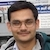# NAEST 2017 Screening Test: Big Thermal SwitchBy

The thermal expansion of materials is a common phenomenon that we fail to see in daily life. The material expansion is usually small and slow that makes it difficult to visualize. This demonstration from NAEST 2017 tries to show this phenomenon.

Question: Select the correct option(s)

1. On heating with candles, the length of the aluminium rod increases.
2. On heating with candles, the length of the aluminium rod decreases.
3. The bulb glows because aluminium rod become conducting on heating.
4. The bulb glows because circuit gets completed due to thermal expansion of aluminium rod.

Solution: An aluminium rod is placed over an array of lighted candles. The heat received from the candles increases its temperature. The length of the rod increases due to an increase in the temperature. The increase in length of a rod of initial length $l_0$ when its temperature increases by $\Delta T$ is given by \begin{align} \Delta l=l_0(1+\alpha\Delta T) \end{align} where $\alpha$ is the coefficient of thermal expansion of the rod's material.

The bulb is connected to a battery as shown in the figure.Initially, the circuit is not complete because the rod's end (point A) is not touching the vertical stand (point B). The switch AB is open. When candles are lighted, the aluminium rod starts expanding due to a rise in its temperature. After some time, the end A touches the end B. The switch AB is closed and the circuit gets completed. The bulb starts glowing at this instant.